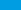ASVAB Math WorksheetsThis is an amazing set of ASVAB prep math worksheets for Arithmetic, Algebra, Geometry & statistics. You can use them for ASVAB preparation.

Need great ASVAB Math worksheets to help you or your students learn basic math concepts in a structured manner? If so, then look no further. This is a perfect and comprehensive collection of FREE printable ASVAB arithmetic reasoning and math knowledge worksheets that would help students in ASVAB Math preparation and practice.

Download our free Mathematics worksheets for the ASVAB test.

Hope you enjoy!

ASVAB Mathematics Concepts

Linear Functions